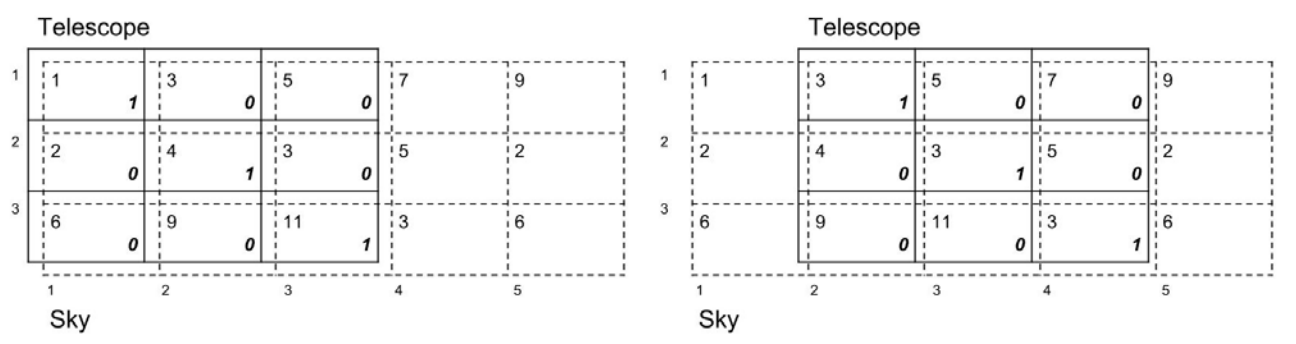시간 제한메모리 제한제출정답맞힌 사람정답 비율
1 초 512 MB65316410724.884%

## 문제

A company named ICPC (Interstellar Cosmology Progress Corporation) is working on a new telescope. It receives optical signals with an m × l grid of sensors. Each sensor detects the color and the intensity of light it receives. Then it filters noises and multiplies the intensity of light by some constant. All the lights sensors received are then merged on a rectangular film. ICPC believes that this telescope can easily beat current optical or radar telescopes because traditional noises are filtered.

However, an engineer found a critical flaw in design. The film can withstand the light with intensity up to W. If it is exposed to the light with intensity greater than W, the telescope may break down.

Consider what will happen in practice.

1. The telescope is on one spot in the sky and as time goes on, it moves to the right. Or, it scans the sky from left to right.
2. The telescope is considered as an m × l grid. The sensor at cell (i, j) of the telescope (1 ≤ i ≤ m, 1 ≤ j ≤ l) amplifies the light it receives by P(i, j) where 0 ≤ P(i, j) ≤ 100.
3. The path where the telescope scans in the sky is an m × n grid. T(i, j) is the intensity of light at cell (i, j) of the path (1 ≤ i ≤ m, 1 ≤ j ≤ n).
4. Initially, the telescope is at position 1 of the path: that is, the sensor at cell (i, j) (1 ≤ i ≤ m, 1 ≤ j ≤ l) of the telescope is aligned with cell (i, j) of the path. Note that one cell of the path is covered by exactly one cell of the telescope.
5. In k minutes, the telescope is at position k + 1 : the sensor at cell (i, j) of the telescope is aligned with cell (i, j + k) of the path.
6. The intensity of light at position k + 1 is Wk = ∑ ∑ T(i, j + k)P(i, j). If Wk > W, then the telescope may break down. Note that the last position of the telescope is n - l + 1.

Consider the following example. The path is a 3 × 5 grid, the telescope is a 3 × 3 grid, and W = 20. Each T(i, j) is written on the left upper side of the grid and each P(i, j) is written on the lower right side of the grid. At position 1, the intensity of light is 1 × 1 + 4 × 1 + 11 × 1 = 16 < W. At position 2, it is 3 × 1 + 3 × 1 + 3 × 1 = 9 < W. You can see that it is smaller than W at position 3 too.Figure L.1: An example of a telescope moving the path from left to right. (left) The telescope is at position 1 of the path. (right) Now the telescope moved to position 2.

Now you want to know how many times the telescope receives light with intensity greater than W, given the information on the sky and the telescope.

## 입력

Your program is to read from standard input. The input starts with a line containing three integers, n (l ≤ n ≤ 10,000), l (2 ≤ l ≤ 3,000), m (2 ≤ m ≤ 100), and W (0 ≤ W ≤ 104 lm), where n is the number of columns in the path, l is the number of columns in the telescope, m is that of rows, and W is the threshold defined above. In the following m lines, information on each row of the path is given line by line. That is, T(i, j) is the jth number of line i + 1. Also, 0 ≤ T(i, j) ≤ 100. In the following m lines, information on each row of the sensors is given line by line. That is, P(i, j) is the jth number of line i + m + 1.

## 출력

Your program is to write to standard output. Print exactly one line for the input. The line should contain the number of times when the intensity of light on the film is greater than W.

## 예제 입력 1

4 2 2 40
1 2 3 4
1 0 0 0
10 10
10 10


## 예제 출력 1

2


## 예제 입력 2

5 3 2 20
1 3 5 7 9
2 4 3 5 2
1 0 0
0 1 0


## 예제 출력 2

0


## 출처

• 데이터를 추가한 사람: gumgood